# Top 10 C++ Interview Questions

Our Subject Matter Experts have produced a collection of C++ interview questions specifically keeping the interview process in mind.

Are you hiring C++ developers? Our Subject Matter Experts have produced a collection of C++ interview questions specifically keeping the interview process in mind. These C++ programming questions will help you conduct face-to-face interviews with prospective hires.

## Question 1

### Look at the following two codes: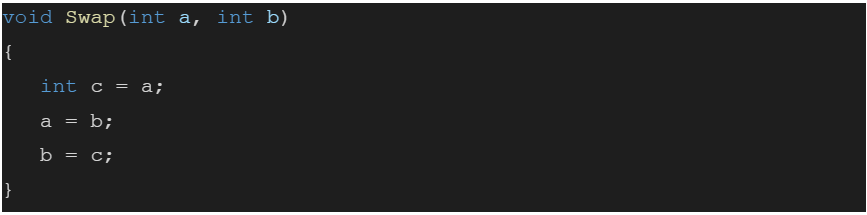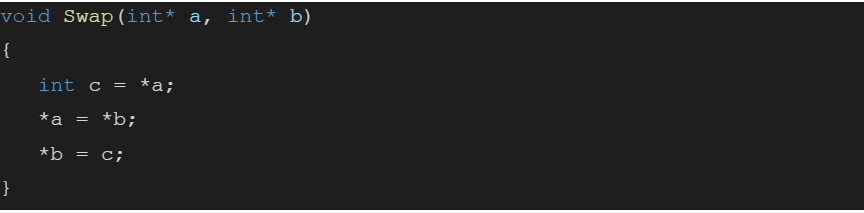What is the difference between the codes used for swapping? Would the first one work? If not, why?

Answer:  The first one is a call-by-value method while the second one is call-by-address (reference) method. In the first method, the values after the call to the Swap() function are stored in local variables a and b. The first Swap() copies the parameters provided in the local variables a and b and then it performs the swap locally. Hence the variable values which are supposed to be swapped do not get swapped at the address (in the RAM). The second code is a call-by-reference function that swaps the values at the address in the RAM.

## Question 2

### What will be the output for the following code? Explain it.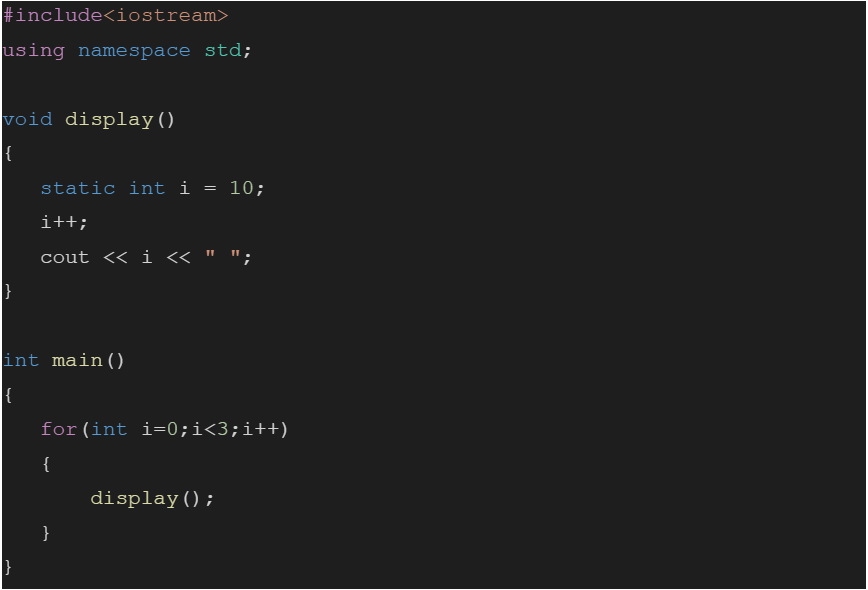Answer: The variable declared in the function display() is a static variable. Static variables preserve their previous value in their previous scope and are not initialized again in the new scope. So the output will be 11 12 13.

## Question 3

### Which of the following two codes is faster and why?

Option A: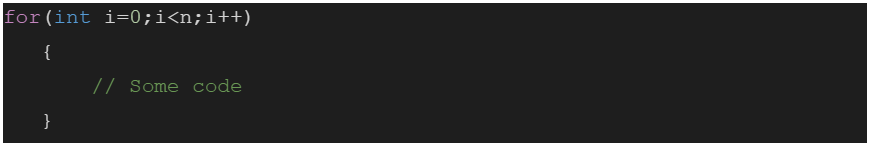Option B: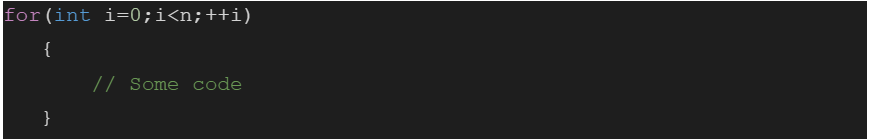Answer: Post-increment usually involves keeping a copy of the previous value around adding a little extra code. Pre-increment simply does it's job and gets out of the way. So, Pre-increment is faster than Post-increment.

## Question 4

### Look at the following code: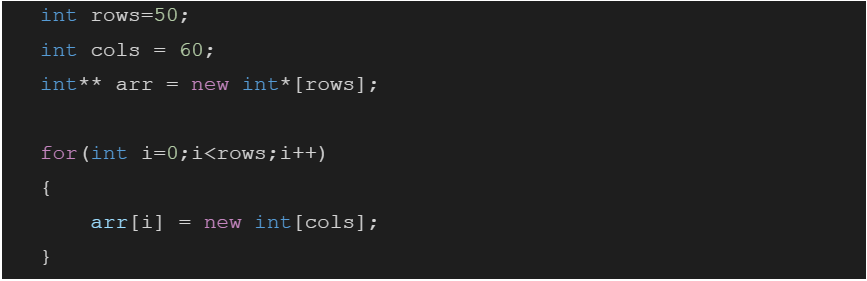How would you deallocate the memory allocated to arr?

Answer: The allocated memory is a 2D array. This memory is allocated in Heap. If we directly deallocate using delete arr, then it would deallocate only the first row and we would lose the pointer to the rest of the rows. So we need to delete each row using delete a[i]. And then delete the main pointer. The following code shows the same: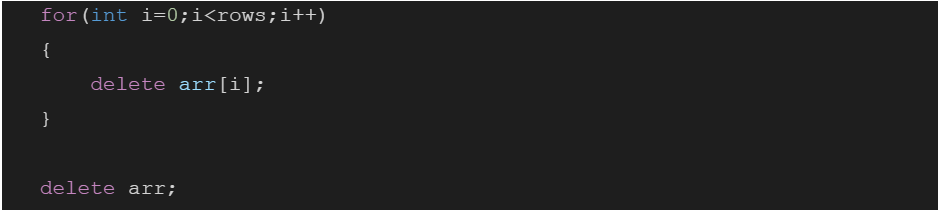## Question 5

### If you are asked to calculate the factorials of large numbers, for eg.75! , how will you calculate it? Just the algorithm. Also, will long long int be sufficient?

Answer: The data type long long is not sufficient for calculating factorials of large numbers. For eg. 100! consists of 158 digits. So it is impossible to store it in a particular data type. Hence, we need an array of digits to store the result.

Algorithm:

1. Factorial(n)

1. Create an array ‘res[]’ of MAX size where MAX is the number of maximum digits in output.
2. Initialize value stored in ‘res[]’ as 1 and initialize ‘res_size’ (size of ‘res[]’) as 1.
3. Do the following for all numbers from x = 2 to n.
• Multiply x with res[] and update res[] and res_size to store the multiplication result.

2. How to multiply a number ‘x’ with the number stored in res[]?

The idea is to use simple school mathematics. We one by one multiply x with every digit of res[]. The important point to note here is digits are multiplied from rightmost digit to leftmost digit. If we store digits in the same order in res[], then it becomes difficult to update res[] without extra space. That is why res[] is maintained in a reverse way, i.e., digits from right to left are stored.

3. multiply(res[], x)

1. Initialize carry as 0.
2. Do following for i = 0 to res_size – 1.
• Find value of res[i] * x + carry. Let this value be prod.
• Update res[i] by storing the last digit of prod in it.
• Update carry by storing remaining digits in carry.

3.   Put all digits of carry in res[] and increase res_size by the number of digits in carry.

## Question 6

### What are Smart Pointers? What important property of C++ OOP(Object Oriented Programming) do they use? How would you implement them?

Answer: Smart pointer is a wrapper class over a pointer with an operator like * and -> overloaded. Just like objects, they get destroyed when they go out of scope, so we don’t have to explicitly call delete().

Implementation: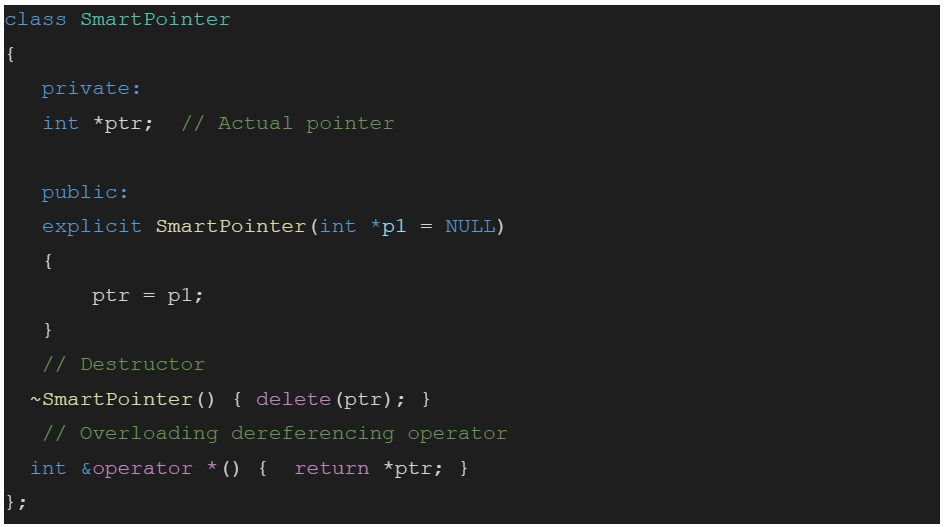## Question 7

### Explain what is an inline function? Will the below code work for calculating Fibonacci numbers? Explain the logic of why it will/won’t work?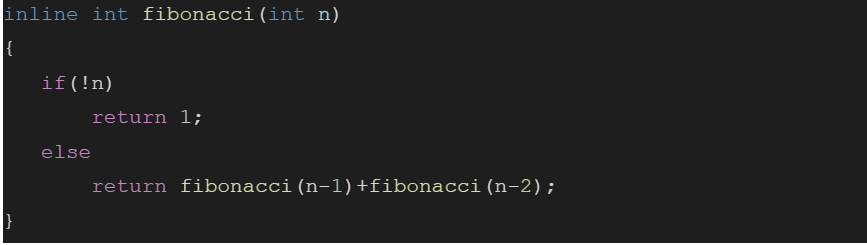Answer: Inline function is a function that is compiled by the compiler as the point of calling the function and the code is substituted at that point. The above function is a recursive function. An inline function cannot be recursive because in case of inline function the code is merely placed into the position from where it is called and does not maintain information on the stack which is necessary for recursion.

## Question 8

### Look at the following codes and conclude how many times the loop will get executed in each example.

Option A: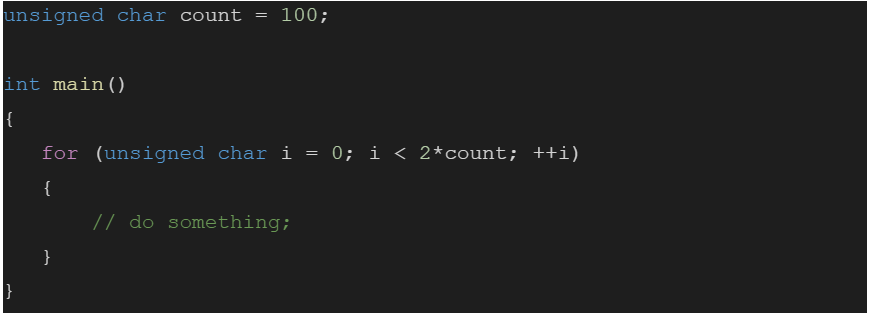Option B: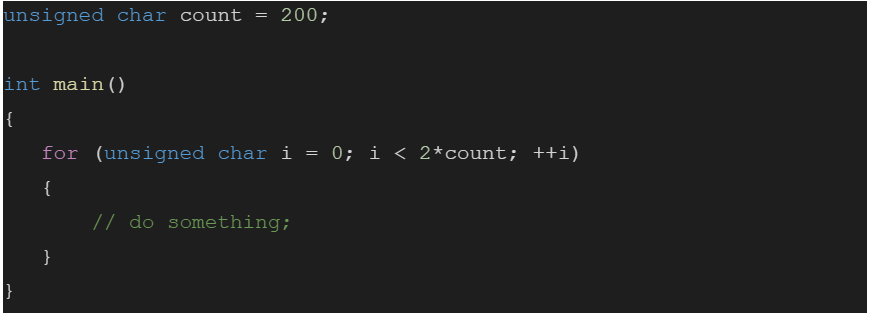Answer: The max value in the case of unsigned char is 255. So in the first case, it will be executed 200 times, while in the second case the counter will overflow after it reaches 255, and it resets to zero. Hence it will result in an overflow. Thus, the second case will be the case of an infinite loop.

## Question 9

### How to print all the odd numbers from 1 to 200 without using for loop or recursion?

Answer: The code is given below.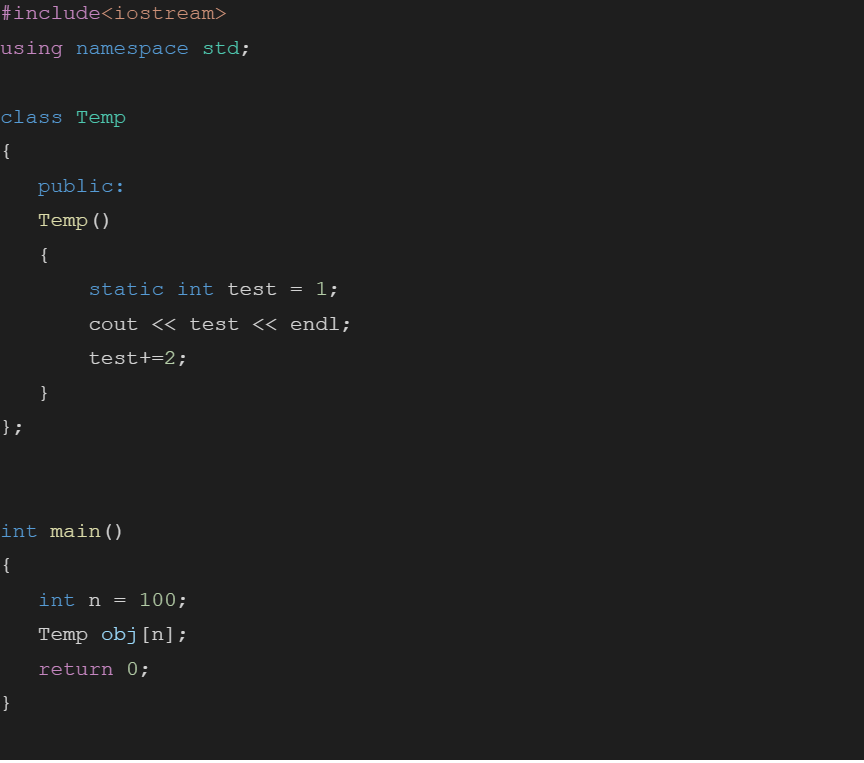## Question 10

### Given two numbers a and b how do you check if the numbers are equal without using ‘==’ operator?

Answer: The code is given below.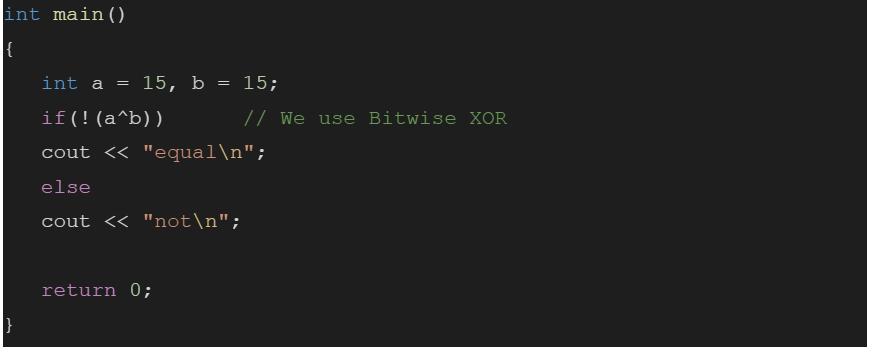Are you hiring C++ developers for your organization? Don't know how to assess them? Check out our C++ online assessments in Basic, Medium and High difficulty modes to screen awesome C++ programmers for your team.

See what candidates are saying about Adaface. Take a peek at our wall of love.